What is a field?

A region of space characterized by a physical property having a determinable value at every point in the region. Examples: gravitational field, Electric field ,and magnetic field

This means if we put anything appropriate in a field, we can then calculate “something” out of that field. In the gravitational field, this “something” is called gravitational force.

Gravitational Field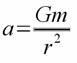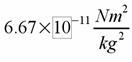A = acceleration

G = gravitational constant                           G=

r = distance to the center of the big object

m = mass of big object

Example: Imagine the pink circle is the Earth and the lines pointing inward are the gravitational field. If we put Bob in the gravitational field, we can calculate the Force acting on him by the Earth. He will follow the gravitational field lines and fall to the surface of the Earth.

(flash can be seen in the power point presentation)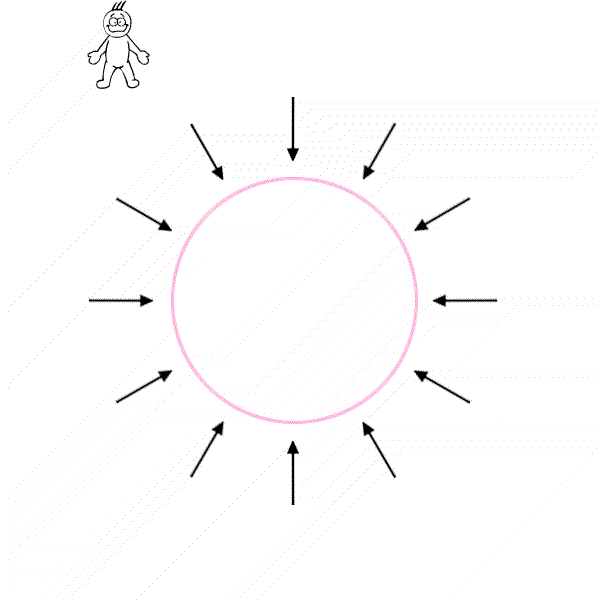Essentially, if we put anything that has a mass in the Gravitational field, we can calculate that “something” I have mentioned before. That “something” is the “gravitational force” in this case. We can determine different kinds of force with different kinds of field.

Electric Field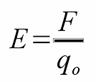E = Electric Field

F = Electric Force

q = Charge

Electric field is defined as the electric force per unit Charge. If we put a +/- (positive or negative) charge in a electric field, the +/- charge will experience a force. This force is called “Electric Force”. (IMPORTANT!!) Recall from G-field. G-field requires an object with a MASS to have a force. E-field requires a +/- charge to have a force. It is the surrounding charges that create an electric field

How do you draw the E-field lines with different charges?

Electric field lines always comes out of the positive charge into the negative charge. Negative charge always absorb the E-field lines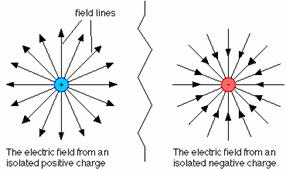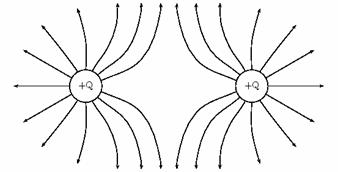What direction is the electric force?

The direction of the Electric force is parallel or tangent to the electric field.

Example: Look at the POINT P on the diagram above (far right). The arrow in the diagram represents the direction of the electric force

Q:             What is the charge, positive or negative, if the direction of the force is pointing outward like in the diagram above?

A:               Positive because it is point away from the stationary positive charge

Q:              What is the charge, if the direction of the force on the diagram switched?

A:               Negative charge

Magnetic FieldB = magnetic field

F = Magnetic Force

Q = charge

V =  velocity of the moving charge

Magnetic field is a field that exerts a force on a moving charge. A magnetic field can be caused either by another moving charge or by a changing of electric field or magnetic dipoles of materials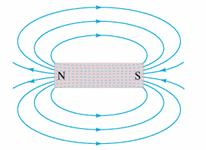How do you draw the magnetic field lines (B-field lines)?

Magnetic field lines come out of N and go into S (see diagram above)

What is the SI units for magnetic field?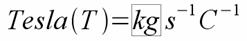The unit of Tesla is a very SMALL unit

- Earth magnetic field has around 10^(-5) Tesla

- A very good Ferromagnet has around 0.5 to 1 Tesla

It is very hard to make material that has a very strong magnetic field

Right Hand Rule

Charge moving in a magnetic field obeys the Right Hand Rule. There are two types of RHR, RHR #1 and RHR #2

Right Hand Rule 1

The thumb represents the velocity of which the + charge (i.e. current) is going. The remaining fingers tell you the direction of the magnetic field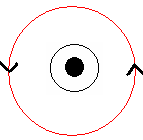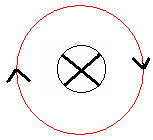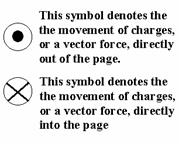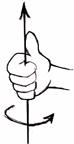Right Hand Rule 2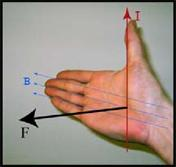I = direction of the charge

B = direction of the Magnetic Field

F = Force act on the charge

Palm Push Positive (*Remember!!)

Examples:

Path of electron moving perpendicular to the magnetic field that is pointing out of the WEBPAGEPath of electron moving perpendicular to the magnetic field that is pointing into the WEBPAGE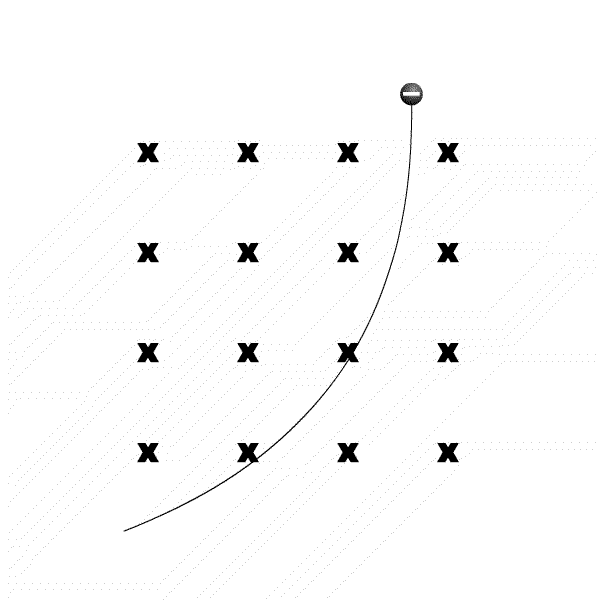Question to ponder

Put a Ferro magnet (N or S) close the turned on CRT from the side.

Note that the electron beam will either go upward or downward

Q: What is the magnetic field (N or S) on the side facing the CRT?

A: It depends on which side you put your magnet close to the CRT, but you can just use RHR #2 to identify the N or S field on the Ferro magnet

Conclusion

The electromagnetic field is a physical field that is produced by electrically charged objects and which affects the behaviour of charged objects. The field can be viewed as the combination of an electric field and a magnet field. The electric field is produced by stationary charges, and the magnetic field by moving charges (currents), changing of the electric field, and dipoles of the material.